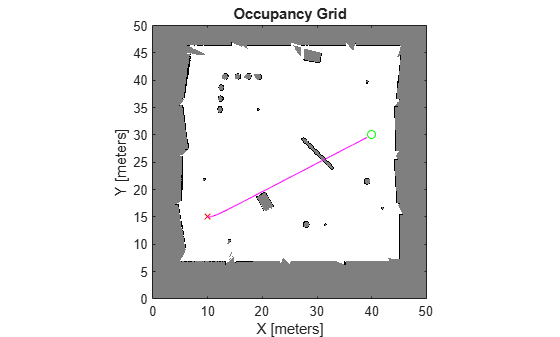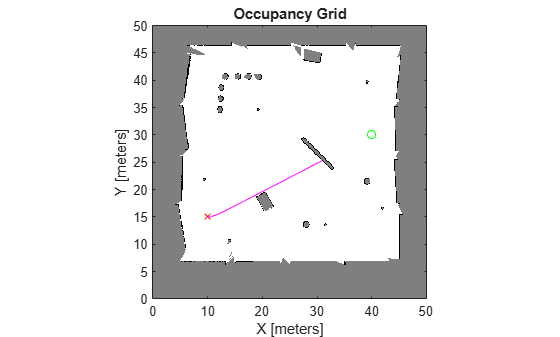# propagate

Propagate system without validation

Since R2021b

## Syntax

``[q,u,steps] = propagate(mobileProp,q0,u0,qTgt,maxSteps)``

## Description

example

``` `[q,u,steps] = propagate(mobileProp,q0,u0,qTgt,maxSteps)` iteratively propagates the system from the current state `q0` towards a target state `qTgt` with an initial control input `u0` for a maximum number of steps `maxSteps`.At the end of each propagation step `i`, the system returns these values: `q(i,:)` — Current state of the system`u(i,:)` — Control input for step ```i + 1````steps(i)` — Number of steps between `i - 1` and `i` ```

## Examples

collapse all

Load a ternary map matrix and create an `occupancyMap` object. Create the state propagator using the map.

```load("exampleMaps","ternaryMap") map = occupancyMap(ternaryMap,10); propagator = mobileRobotPropagator(Environment=map);```

Set the state bounds on the state space based on the map world limits.

```propagator.StateSpace.StateBounds(1:2,:) = [map.XWorldLimits; map.YWorldLimits];```

Specify the start and goal states.

```start = [10 15 0]; goal = [40 30 0];```

Specify the initial control and maximum number of steps.

```u0 = [0.2 -0.2]; maxSteps = 2000;```

Propagate the state of the robot without validation.

`[q1,u1,steps1] = propagate(propagator,start,u0,goal,maxSteps);`

Visualize the results.

```figure show(map) hold on plot(start(1),start(2),"rx") plot(goal(1),goal(2),"go") plot(q1(:,1),q1(:,2),"m") hold off```Propagate the state of the robot and return valid motion.

`[q2,u2,steps2] = propagateWhileValid(propagator,start,u0,goal,maxSteps);`

Visualize the results.

```figure show(map) hold on plot(start(1),start(2),"rx") plot(goal(1),goal(2),"go") plot(q2(:,1),q2(:,2),"m") hold off```## Input Arguments

collapse all

Mobile robot state propagator, specified as a `mobileRobotPropagator` object.

Initial state of the system, specified as an s-element vector. s is the number of state variables in the state space.

Initial control on the initial state, specified as an c-element vector. c is the number of control inputs.

Target state of the system, specified as an s-element vector. s is the number of state variables in the state space.

Maximum number of steps, specified as a positive scalar.

## Output Arguments

collapse all

Propagated states of the system, returned as an s-element vector. s is the number of state variables in the state space.

Control inputs for propagating states, returned as an n-by-c matrix. c is the number of control inputs

Number of steps from each state and control input to next, returned as an n-element vector of positive integers.

## Version History

Introduced in R2021b